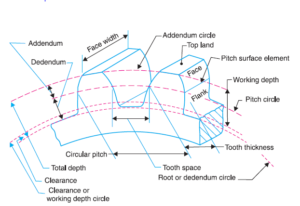## Gear Ratio: Definition, Formula, Advantages, Uses

Gear Ratio The gear ratio of a gear train is the ratio of the angular velocity of the input gear to the angular velocity of the output gear, also known as the speed ratio of the gear train. The gear ratio can be computed directly from the numbers of teeth of the...